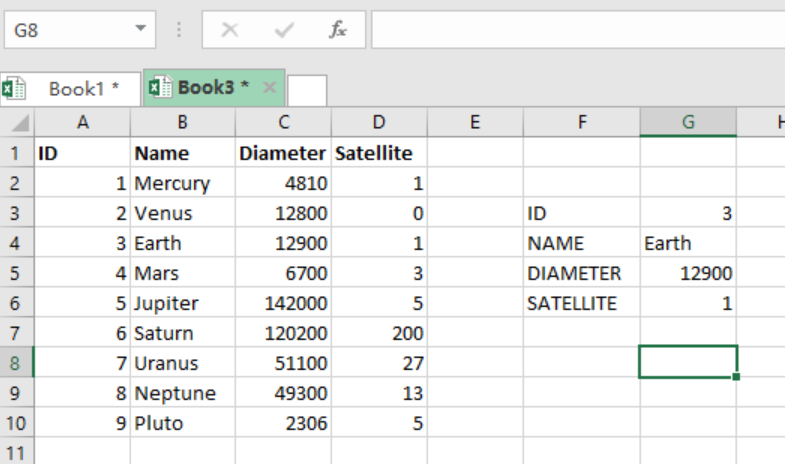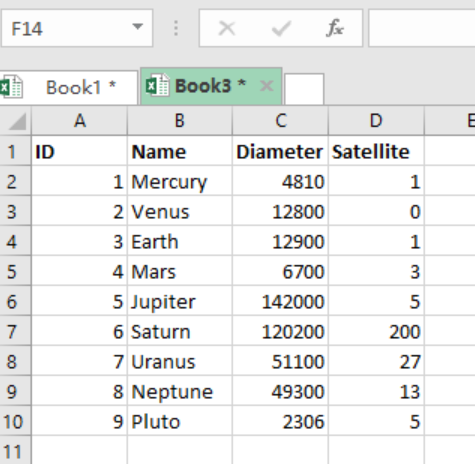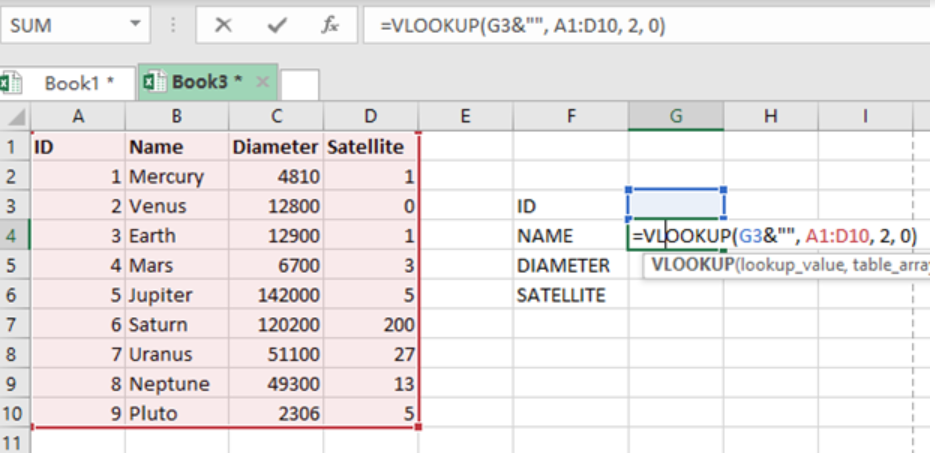Get instant live expert help with Excel or Google Sheets“My Excelchat expert helped me in less than 20 minutes, saving me what would have been 5 hours of work!”

#### Post your problem and you’ll get Expert help in seconds.

Your message must be at least 40 characters
Our professional Expert are available now. Your privacy is guaranteed.

# How to Use VLOOKUP with Numbers and Text

If we want to use the VLOOKUP function with both numbers and text, there usually is a mismatch between numbers and text. This article will step through the process of successfully using the VLOOKUP function with number and text.Figure 1. Final result

## Formula

Below are the original and revised forms of the formula.

`=VLOOKUP(id, planets, 2, 0) // Original`

`=VLOOKUP(id &””, planets, 2, 0) // Revised`

## How the function works

Concatenating an empty string to a number converts it to text. This can also be done with a longer formula which utilizes the TEXT function to convert to text.

`=VLOOKUP(TEXT(id, “@”), planets, 2, 0)`

Even with these formulas, we might still be unsure whether we are dealing with numbers or text. In such an instance, we need to wrap the VLOOKUP formula inside IFERROR. This will yield the formula below:

`=IFERROR(VLOOKUP(id, planets, 3, 0), VLOOKUP(id&””, planets, 3, 0))`

## Example 1: VLOOKUP with numbers and text

Step 1: Prepare the dataFigure 2. Example on how to use VLOOKUP with Numbers and Text

Step 2: Specify where to put the formula then Press Enter.Figure 3. Example on how to use VLOOKUP with Numbers and Text

Your final result should be as below:Figure 4. Example on how to use VLOOKUP with Numbers and Text

Instant Connection to an Expert through our Excelchat Service

Most of the time, the problem you will need to solve will be more complex than a simple application of a formula or function. If you want to save hours of research and frustration, try our live Excelchat service! Our Excel Experts are available 24/7 to answer any Excel question you may have. We guarantee a connection within 30 seconds and a customized solution within 20 minutes.

Are you still looking for help with the VLOOKUP function? View our comprehensive round-up of VLOOKUP function tutorials here.

### Did this post not answer your question? Get a solution from connecting with the expert.Another blog reader asked this question today on Excelchat:
Solution exampleshi, my vlookup wont work in some rows but does in others. its temperemental. e.g it will work on rows 10 to 15, then not row 16 and 17, but the will for the rest.
Solved by K. Q. in 21 minsI have a pivot table in which per order (on the rows) stands how much products they ordered per size (on the columns). I want to determine which combinations of quantities of sizes people order. And I want to count these combinations.
Solved by E. W. in 60 minsI need a formula to check a short list of names and put the appropriate value for that name in. For example, "Supermarket 1" and the value "20%" and "Supermarket 2" with "30%", so each time Column A has "Supermarket A" written in a cell, the appropriate cell in Column B will autofill with the right value instead of having to consult the list and manually enter it each time. This value will then be checked by later formulae
Solved by E. J. in 37 minsI have 500 numbers in column A with aproximatly 15digits. In column B I have 5 numbers with 6 digits Can a find a formula wich can transfer all numbers from A column wich start with some 6 digitsa from B column
Solved by V. L. in 32 minsI have a sheet with 3 columns. First column is a code for records in column B (has 563 records). What I need is a formula to show me if what is in column C (has 4400 records) is in column B and if it is to take the code for that record. Example: Column A(code corresponding to column B): 12, 14, 15, 19 Column B(names): asd, adf, ade, aqw Column C(names): akd, adf, ade, anb, wgs I need something that would say, if record in column C (for example adf) is matching record in column B (adf) return the code from column A (the code corresponding to that record in column B),if not return blank.
Solved by C. J. in 8 mins## Subscribe to Excelchat.coAnother blog reader asked this question today on Excelchat: